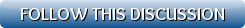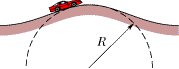[Show all top banners]
 Back to: Kurakani General Discussion Refresh page to view new replies[VIEWED 4407 TIMES] SAVE! for ease of future access.
nepwantedPosted on 05-29-09 6:42 PM     Reply [Subscribe]
Login in to Rate this Post:     0?A box of canned goods slides down a ramp from street level into the basement of a grocery store with acceleration 0.55 m/s2 directed down the ramp. The ramp makes an angle of 40Â° with the horizontal. What is the coefficient of kinetic friction between the box and the ramp?

n the figure below, a stuntman drives a car (without negative lift) over the top of a hill, the cross section of which can be approximated by a circle of radius R = 170 m. What is the greatest speed at which he can drive without the car leaving the road at the top of the hill?
hpilot23Posted on 05-29-09 8:03 PM     Reply [Subscribe]
Login in to Rate this Post:     0?haha yo question exam ma aaunewala cha hoo babu???...lol..

vikingsPosted on 05-29-09 8:46 PM     Reply [Subscribe]
Login in to Rate this Post:     0?mass*acceleration=mass*gsin(45)-coeff*mass*gcos(45)

Last edited: 30-May-09 12:46 AM

nepaleanPosted on 05-29-09 9:17 PM     Reply [Subscribe]
Login in to Rate this Post:     0?यो त सार्है सजिलो भएन र गुरुकै किन खोजी गर्नुपर्यो चेलाले पनि जान्लान नि। हुन त धेरै भो यस्ता हिसाब नदेखेको , त्यही पनि ट्राइ मारुम है त?
१) F= W*Sin40
W*Sin40-f=Ma
W*Sin40-uN=Ma
W*Sin40-uWcos40=Ma
W = weight,u=coeff frction, a=acceleration, M=mass
answer should be around 0.76 आंफै गरु है।

nepaleanPosted on 05-29-09 9:24 PM     Reply [Subscribe]
Login in to Rate this Post:     0?हो कि हो कि जस्तो लाग्छ यो पनि।
Cforce=W-N
mv^2/r=W-N
N=0 भए गाडी खस्छ
mv^2/r=mg-0
answer should be around 7.081 आंफै गरु है।

nepwantedPosted on 05-29-09 10:07 PM     Reply [Subscribe]
Login in to Rate this Post:     0?nepalean chai guru nai ho rahecha...... Thanks dude.......

Pilot bro, exam ma aaune bhaye ta prof lai nai sodhthe ni......

Actually it was webassign H/W. I could not figure out these two and i am at work. Couldn't give more time. Thanks nepalean.. First one is right.

KalubhaiPosted on 05-30-09 12:47 AM     Reply [Subscribe]
Login in to Rate this Post:     0?For the Second part, I think answer should be as follows:
f = (V^2/ 15R) - 0.01 e
where,
f = Side friction, which depends on road type, condition and speed of vehicle
V = Speed in mph, R = Radius in ft
Since f depends on speed as well, the solution should be in quadratic form.
Suppose, e =0 (no superelevation)

Speed (mph)             f-value
10                             0.21
20                             0.21
30                             0.18
40                             0.16
50                             0.14
60                             0.13
70 & more                 take 0.10

So, pick a f value, calculate speed and check if it matches for your speed taken for that f-value.
(I forgot equation for f -value)

For Example:
e =0, f=0.17, R = 558 ft (170m)
Hence, V= 37 mph, and for this speed f=0.17

naivelyStupidPosted on 05-30-09 2:31 AM     Reply [Subscribe]
Login in to Rate this Post:     0?My common sense says

a)  Coeff.  * g cos 40 = g sin 40 - 0.55

b) V_max ^2 / R = g   (they're talking about the top point)

CEOPosted on 05-30-09 3:59 PM     Reply [Subscribe]
Login in to Rate this Post:     0?Newton's First Law: Qualitative definition of Force. If no NET EXTERNAL FORCE acts on an object, the object will neither change direction nor its speed, nor both.

Start: What is the net external force in your problem?

What are the forces acting on the object? Gravity and Friction.

Check: Are they acting on the same direction? Yes or No.

In this case: No.

If No, then are they acting perpendicular to each other? No.

If No, then the component of the Gravity and Friction in the direction of motion (in this case sliding). Component of gravity parallel to direction of motion = Weight * sin_theta (where theta is the  angle the motion surface makes with horizontal).

Friction is acting parallel to the surface of the motion, but always acts against (opposite) the motion. So, it is mu_k(coef. of kinetic friction) * component of force normal to the surface of motion.

The component of force normal to the surface of motion = Weight * cos_theta (where theta is the  angle the motion surface makes with horizontal).

Net external force (=net driving force) = difference between aforemention two forces = Gravity component in the direction of motion - friction force on the surface of motion(note it always acts against motion) = W * sin_theta - mu_k* W*cos_theta

Now, what is second law of motion (Newton's second law). Force = mass * acceleration.  Similarly, apply newton's third law of motion: action (= net driving force)  = reaction (i.e. mass * acceleration)

So, mass* acceleration = driving force =  W * sin_theta - mu_k* W*cos_theta  (replace W by mass*g )

So, mass* acceleration = mass*g * sin_theta - mu_k* mass*g *cos_theta

cancel mass from the equation because it is common.

Solve this equation for mu_k (coefficient of friction) = (g * sin_theta - acceleration )/ (g *cos_theta)

You are done. Note, if you get mu_k negative, then what is your interpretation? As I said above, friction always acts against the motion. You must think it and make you interpretation correctly. Friction is a non-conservative force. If you get negative coefficient of friction, the net driving force is not enough to counter the friction, and therefore, there is acceleration, but decceleration. If the object is not in motion as an initial condition, then you will know know kinetic friction. Your answer is "not enough information". Period.